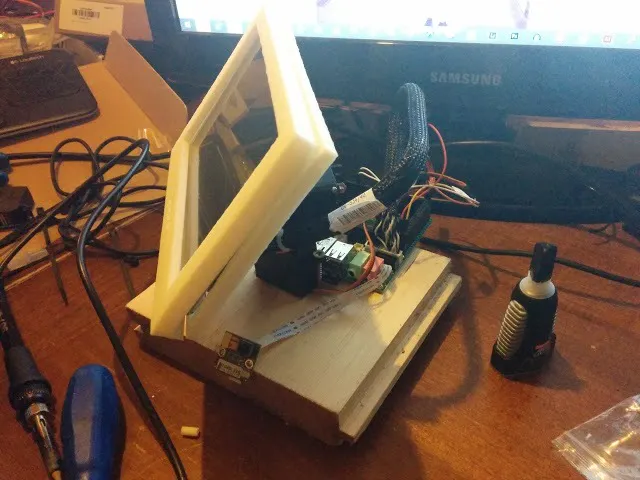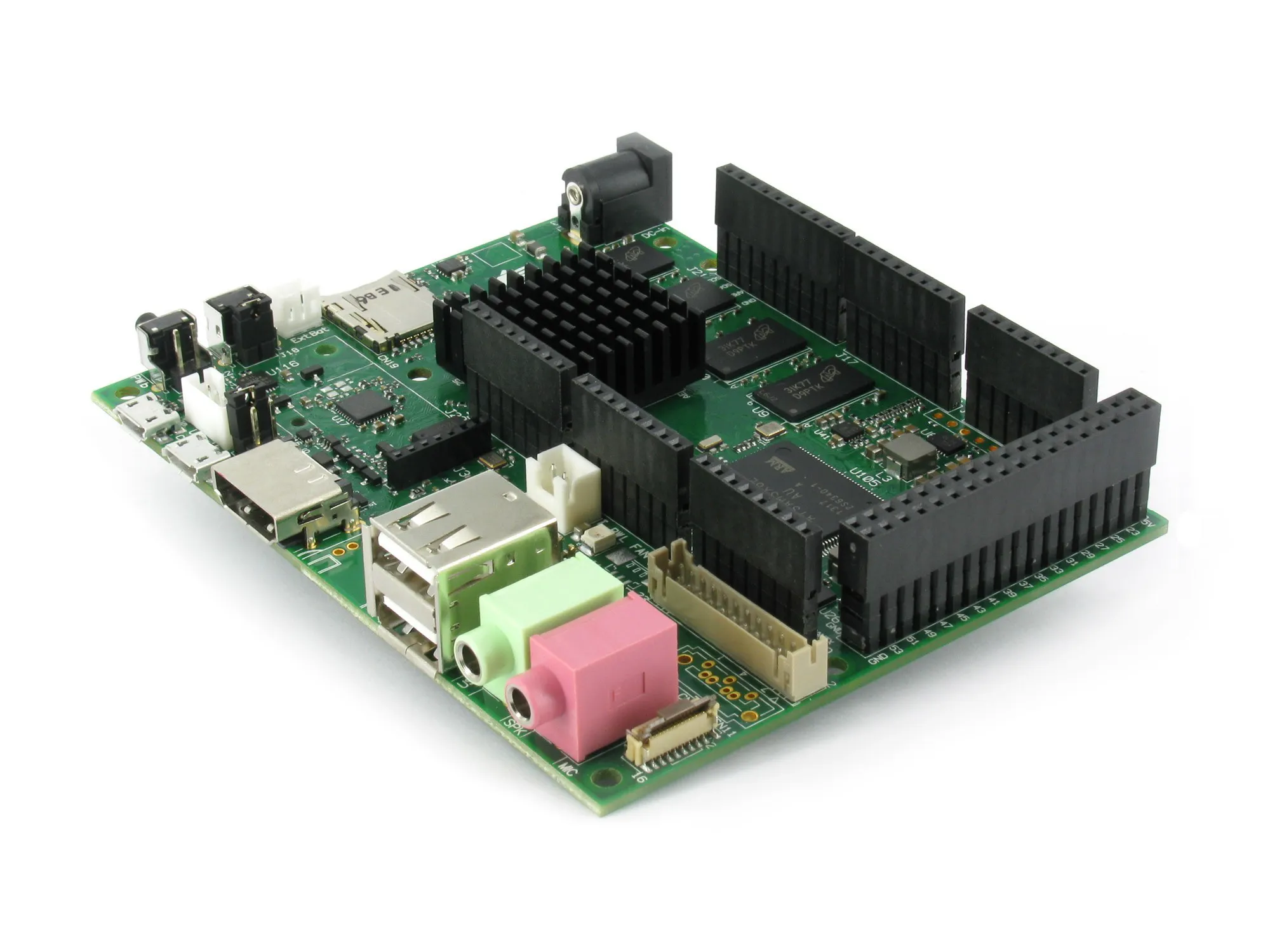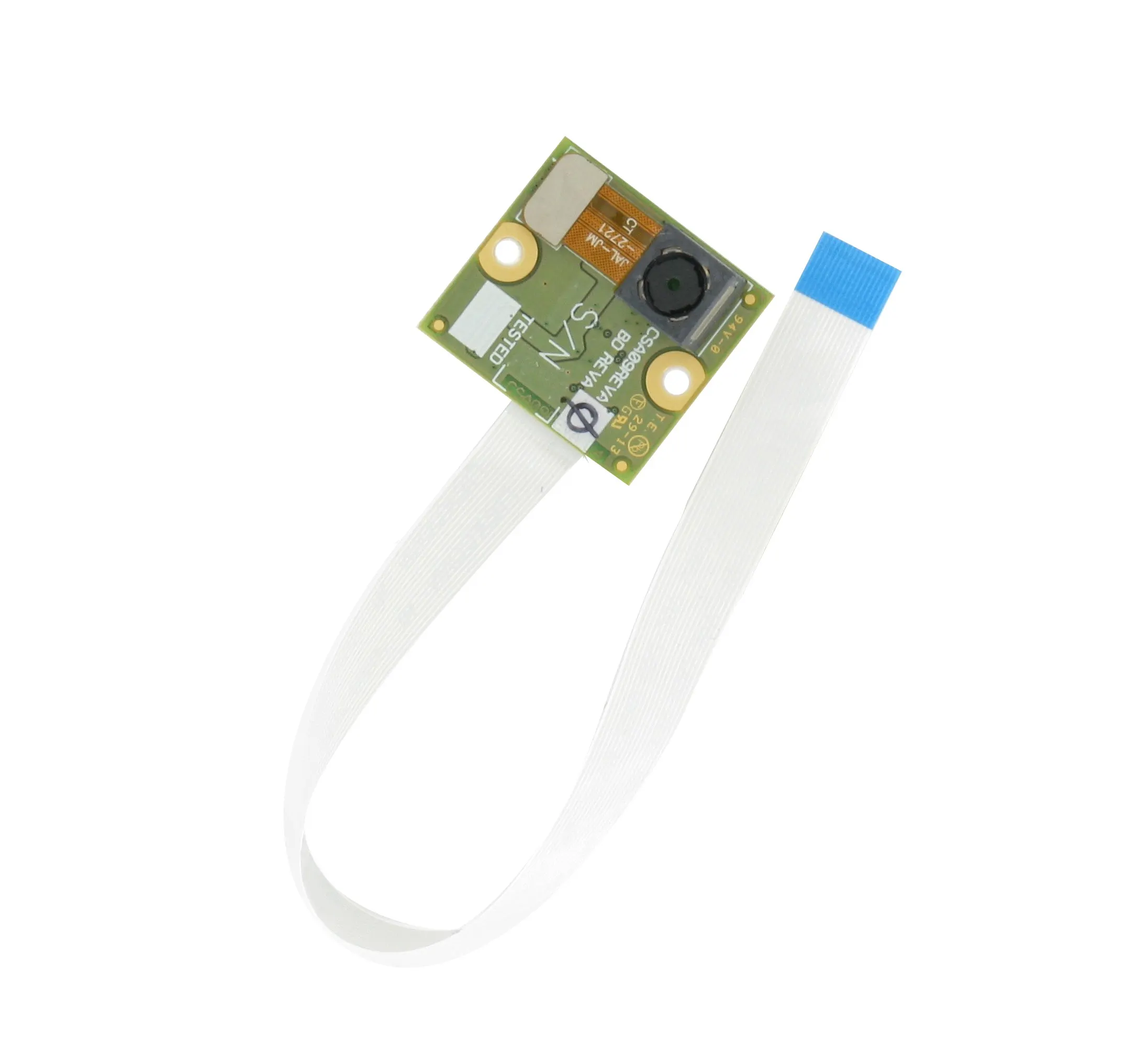Published

# Face Recognition & Face Tracking via OpenCV and UDOO

Make a screen move towards your face when it moves in the camera range

Full instructions provided6,475## Things used in this project

### Hardware componentsUDOO DUAL
×1
 MicroSD with Ubuntu pre-installed
×1UDOO Camera Module or Compatible USB Camera
×1
 UDOO Screen
×1
 Servos (Tower Pro MG996R)
×2

### Software apps and online servicesOpenCV

## Code

### code.txt

Plain text
```sudo pip install pyserial
```

### code.txt

Python
```import cv2
import sys
import serial

# Create an object Serial with ttymxc3 port
ser = serial.Serial(
port='/dev/ttymxc3',
baudrate=115200,
parity=serial.PARITY_ODD, # Optional
stopbits=serial.STOPBITS_ONE, # Optional
bytesize=serial.EIGHTBITS # Optional
)

# Check if Serial is Open, close it then re-open (to avoid exception)
if ser.isOpen():
ser.close()

ser.open()
cascPath = sys.argv

# Set video source (UDOO Camera) and resolution
video_capture = cv2.VideoCapture(7)
video_capture.set(3,320)
video_capture.set(4,240)

while True:
# Capture frame-by-frame
gray = cv2.cvtColor(frame, cv2.COLOR_BGR2GRAY)
gray,
scaleFactor=1.2,
minNeighbors=5,
minSize=(30, 30),
flags=cv2.cv.CV_HAAR_SCALE_IMAGE
)

# Draw a rectangle around the faces
for (x, y, w, h) in faces:
print ("x: ",x,", y: ",y)
prov=str(x)
prov = prov + 'x'
ser.write(prov)
prov=str(y)
prov = prov + 'x'
ser.write(prov)
cv2.rectangle(frame, (x, y), (x+w, y+h), (0, 255, 0), 2)

# Display the resulting frame
cv2.imshow('Video', frame)

if cv2.waitKey(1) & 0xFF == ord('q'):
break

# When everything is done, release the capture
video_capture.release()
cv2.destroyAllWindows()
```

### code.txt

C/C++
```#include

int data, v;
int i=0, j=0, n=0, prov=320, num, plus=320, plus1=240, aum=45, aum1=45;
char c;
Servo servo1;
Servo servo2;

void setup()
{
servo1.attach(2);
servo2.attach(3);
Serial.begin(9600);
}

void loop()
{
//Read Serial bus data received from Python and write them into servo position
if(Serial.available())
{
if(prov!=72)
{
data[i]=prov;
i++;
}
else
{
if(i==0)
num=0;
if(i==1)
num=data;
if(i==2)
num=data+data*10;
if(i==3)
num=data+data*10+data*100;
i=0;
v[n]=num;
n++;
if(n==2)
{
//Start X axis
while(plus>0)
{
if((v<=plus) && (v>(plus-10)))
{
servo1.write(aum-5);
delay(15);
plus=0;
}
else
{
plus=plus-10;
aum=aum+3;
}
}
plus=320;
aum=45;
//Start Y axis
while(plus1>0)
{
if((v<=plus1) && (v>(plus1-10)))
{
servo2.write(aum1);
delay(15);
plus1=0;
}
else
{
plus1=plus1-10;
aum1=aum1+3;
}
}
plus1=240;
aum1=45;
n=0;
}
}
}
}
```

### code.txt

Plain text
```sudo python file_name.py xml_name.xml
```

### code.txt

Plain text
```sudo python webcam.py haarcascade_frontalface_default.xml
```

### Github

https://github.com/MakerMark/FaceTracking

## Credits

### Marco Mancini

2 projects • 13 followers
#Maker, student and beer consumer at @UDOO_Board dev team. Founder of #GDGCamerino, the @google dev group of my own town. Love #robotics, #DIY, #Drones.